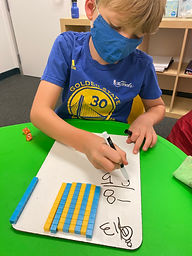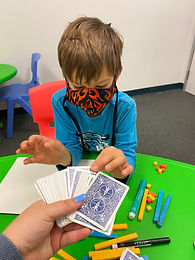## Ms. Briana

### Target 1​

###### Lesson Type:

Continuation

Number Operation

:

Number Reltionships

Compare equations, recording the results of the comparison with the symbols >, =, and <.

2nd

###### Vocabulary:

Greater than, Lesser Than, Equal, Place Value, Symbols and Comparison

Activities:

Students used spinning wheel to create basic subtraction equations to compare.

Students then got to choose which manipulative they wanted to use to create a Variety of equations for them to compare .### Home Exploration

###### Guiding Questions:## Absent Students:

### Target 2

:

###### 1:

Understand why the standard algorithm for subtraction is based on place-value.

###### 2:

Understand that when subtracting a multi-digit number, each place-value is being subtracted individually to create the new number (ones are subtracted from ones, tens are subtracted from tens, etc.)

###### 3:

Understand that when adding a multi-digit number, to solve start in the lowest place-value and go left.

###### 4:

Understand how to regroup based on place-value understandings (i.e., ten ones can be composed into one ten – ten tens can be composed into one hundred).

2nd

###### Vocabulary:

Multi-digit, Standard form, Place Value, Difference and Regrouping

Activities:

Students used 10 sided dice to create double digit subtration problems to solve.

Students used playing cards to create double digit cubtraction problems to solve.### Home Exploration

###### Guiding Questions:### Target 3

:

###### Vocabulary:

Activities:### Home Exploration